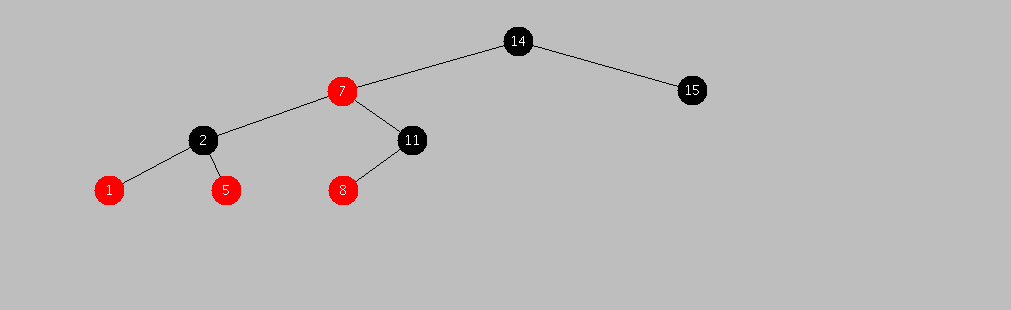# Purely Functional Data Structures & Algorithms : Red-Black Trees in Qi

Update 2011/06/28 : Source has been modified to compile with Shen

This is the first in a series of posts that will demonstrate the implementation of many well-known(and less known) data structures and algorithms using a purely functional approach.
We will use Qi as our implementation language for a number of reasons :

It’s a Lisp : macros, EVAL, hash-tables, property-lists, meta-programming etc.
Pattern matching.
Optional static type checking.
A Turing-complete type system !

In this first post we look at an implementation of the well-known Red-Black tree abstract data type in Qi.

A red–black tree is a type of self-balancing binary search tree, a data structure used in computer science, typically to implement associative arrays. The original structure was invented in 1972 by Rudolf Bayer and named “symmetric binary B-tree,” but acquired its modern name in a paper in 1978 by Leonidas J. Guibas and Robert Sedgewick. It is complex, but has good worst-case running time for its operations and is efficient in practice: it can search, insert, and delete in O(log n) time, where n is total number of elements in the tree. Put very simply, a red–black tree is a binary search tree that inserts and removes intelligently, to ensure the tree is reasonably balanced.Our implementation comes in at 57 lines of code (with the balance function at only 7 lines)

```(tc +)
(datatype tree-node
Key : number; Val : B;
======================
[Key Val] : tree-node;)
(datatype color
if (element? Color [red black])
_______________________________
Color : color;)
(datatype tree
if (empty? Tree)
________________
Tree : tree;
Color : color; LTree : tree; TreeNode : tree-node; RTree : tree;
================================================================
[Color LTree TreeNode RTree] : tree;)
(define node-key
{tree-node --> number}
[Key Val] -> Key)
(define make-tree-black
{tree --> tree}
[Color A X B] -> [black A X B])
(define member
{tree-node --> tree --> boolean}
X NIL -> false
X [Color A Y B] -> (if (< (node-key X) (node-key Y))
(member X A)
(if (< (node-key Y) (node-key X))
(member X B)
true)))
(define balance
{tree --> tree}
[black [red [red A X B] Y C] Z D] -> [red [black A X B] Y [black C Z D]]
[black [red A X [red B Y C]] Z D] -> [red [black A X B] Y [black C Z D]]
[black A X [red [red B Y C] Z D]] -> [red [black A X B] Y [black C Z D]]
[black A X [red B Y [red C Z D]]] -> [red [black A X B] Y [black C Z D]]
S -> S)
(define insert-
{tree-node --> tree --> tree}
X [] -> [red [] X []]
X [Color A Y B] -> (if (< (node-key X) (node-key Y))
(balance [Color (insert- X A) Y B])
(if (< (node-key Y) (node-key X))
(balance [Color A Y (insert- X B)])
[Color A Y B])))
(define insert
{tree-node --> tree --> tree}
X S -> (make-tree-black (insert- X S)))
```

This is a reasonably performant implementation (we haven’t even tried to optimize it yet).

```(19-) (run-tests NIL)
tree: [black
[red [black [red [] [1 1] []] [2 2] [red [] [5 5] []]] [7 7]
[black [red [] [8 8] []] [11 11] []]]
[14 14] [black [] [15 15] []]]
12 is a member ? false
8 is a member ? true
Creating tree with 100000 elements ...
Evaluation took:
0.578 seconds of real time
0.562833 seconds of total run time (0.491572 user, 0.071261 system)
[ Run times consist of 0.160 seconds GC time, and 0.403 seconds non-GC time. ]
97.40% CPU
1,210,617,335 processor cycles
168,551,696 bytes consed
Performing lookups in tree with 100000 elements ...
666 in tree ? true
Evaluation took:
0.000 seconds of real time
0.000044 seconds of total run time (0.000035 user, 0.000009 system)
0.00% CPU
86,110 processor cycles
0 bytes consed
-1 in tree ?
Evaluation took:
0.000 seconds of real time
0.000024 seconds of total run time (0.000021 user, 0.000003 system)
100.00% CPU
46,368 processor cycles
0 bytes consed
```

A comparable implementation in Java/C++ will usually run a few hundred lines of code.
All Qi code in this post is here.